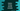# Python numpy.around() method explanation with example## Python numpy around method:

The around() is an inbuilt method of numpy that can be used to evenly round the content of an array to a given number of decimals. In this post, we will learn how to use numpy around() with its definition and examples.

### Definition of around():

numpy.around() method is defined as like below:

``numpy.around(arr, decimals=0, out=None)``

Here,

• arr is the input array_like data
• decimals is the number of decimal places to round. It is an optional value and we can also provide a negative value.
• out is also an optional value. It is an array to put the result. It must have the same shape as the output expected. But the type will be cast if required.

### Return value of around():

around() returns a new array of the same type as the input array.

### Example of numpy.around():

Let’s take an example:

``````import numpy as np

arr = [1, 2.5, 2.6, 3.0, -2.6]

result = np.around(arr)

print('Given array: ', arr)
print('Final array: ', result)``````

It uses numpy.around() with the array arr and the result is stored in the result variable.

It will print:

``````Given array:  [1, 2.5, 2.6, 3.0, -2.6]
Final array:  [ 1.  2.  3.  3. -3.]``````

### Example of numpy.around() with a different decimal value:

The above example uses 0 as the decimal. We can also provide any other decimal value. The below example uses 3 as the decimal.

``````import numpy as np

arr = [1, 2.5234, 2.6122, 3.0555, -2.656565]

result = np.around(arr, decimals=3)

print('Given array: ', arr)
print('Final array: ', result)``````

It will print:

``````Given array:  [1, 2.5234, 2.6122, 3.0555, -2.656565]
Final array:  [ 1.     2.523  2.612  3.056 -2.657]``````

### Example of numpy.around() with a negative decimal value:

We can pass a negative value as decimal. For negative values, it specifies the number of positions to the left of the decimal point.

For example:

``````import numpy as np

arr = [1, 90992.5234, 22342.6122, 3554.0555, -223.656565]

result = np.around(arr, decimals=-3)

print('Given array: ', arr)
print('Final array: ', result)``````

It will give:

``````Given array:  [1, 90992.5234, 22342.6122, 3554.0555, -223.656565]
Final array:  [    0. 91000. 22000.  4000.    -0.]``````

### Pass the array as its argument:

In the above examples, we are storing the return value of np.around() in a new variable. Instead, we can also pass the array as one of its argument. It must have the same shape as the output.

``````import numpy as np

arr = [1, 90992.5234, 22342.6122, 3554.0555, -223.656565]
result_arr = np.empty((1, 5))

np.around(arr, decimals=-3, out=result_arr)

print('Given array: ', arr)
print('Final array: ', result_arr)``````

It will give similar result.